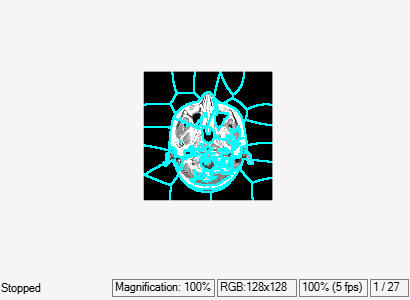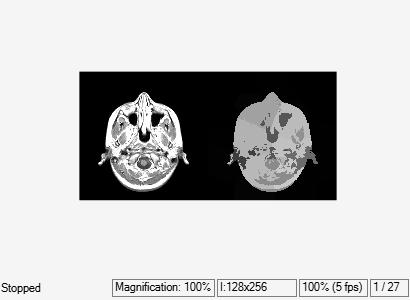# Compute 3-D Superpixels of Input Volumetric Intensity Image

Load 3-D MRI data, remove any singleton dimensions, and convert the data into a grayscale intensity image.

```load mri; D = squeeze(D); A = ind2gray(D,map); ```

Calculate the 3-D superpixels. Form an output image where each pixel is set to the mean color of its corresponding superpixel region.

```[L,N] = superpixels3(A,34); ```

Show all xy-planes progressively with superpixel boundaries.

```imSize = size(A); ```

Create a stack of RGB images to display the boundaries in color.

```imPlusBoundaries = zeros(imSize(1),imSize(2),3,imSize(3),'uint8'); for plane = 1:imSize(3) BW = boundarymask(L(:, :, plane)); % Create an RGB representation of this plane with boundary shown % in cyan. imPlusBoundaries(:, :, :, plane) = imoverlay(A(:, :, plane), BW, 'cyan'); end implay(imPlusBoundaries,5) ```Set the color of each pixel in output image to the mean intensity of the superpixel region. Show the mean image next to the original. If you run this code, you can use `implay` to view each slice of the MRI data.

```pixelIdxList = label2idx(L); meanA = zeros(size(A),'like',D); for superpixel = 1:N memberPixelIdx = pixelIdxList{superpixel}; meanA(memberPixelIdx) = mean(A(memberPixelIdx)); end implay([A meanA],5); ```﻿